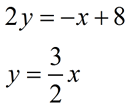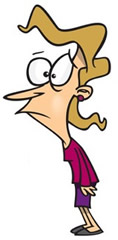Practice with Systems of Equations Terms of Use    Contact Person: Donna RobertsDirections: You may need paper and pencil. Choose the correct answer.

1.
A system of equations is given as:
x = 8
3x + 4y = 16
What is the value of y?

Choose:
 -2 2 -10 10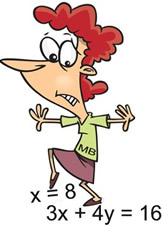2.
Given the system:
3x + 2y = 13
x - 2y = 3
What is the solution to this system?

Choose:
 (-4, ½) (4, ½) (2½, ¼) (-2½, ¼)3.
Given the system:
2x + 3y = 21
x + y = 3
What is the solution to this system?Choose:
 x = 18     y = -15 x = 2     y = 1 x = -12     y = 15 x = 12     y = -9

4.
Given the system:
4x - y = 10
2x = 12 - 3y
What is the solution to this system?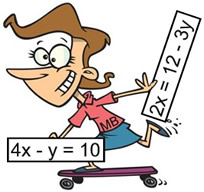Choose:
 (3, -2) (3, 2) (4, 6) (-3, -22)

5.
Given the system:
x - 2y = -7
x - y = -4
What is the solution to this system?Choose:
 (-1, 3) (1, -3) (-1, -3) (-1, -3)

 6. Given the following system of equations:       y = 3x + 4       y = 8x + 4 Which of the following statements are TRUE about this system of equations? Check all that apply, and hit SUBMIT! The system has exactly one solution. The system has no solution. The graph of the system are straight lines that intersect in exactly one location. The graph of the system are straight lines that do not intersect. The lines of this system intersect on the x-axis. The lines of this system intersect on the y-axis.

7.
Given the following equations:
Y1 = ¾ x - 4
Y2 = -x + 3

Which of the following graphs correctly shows the solution of this system?

Choose: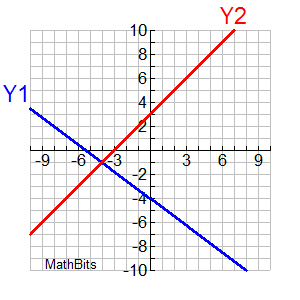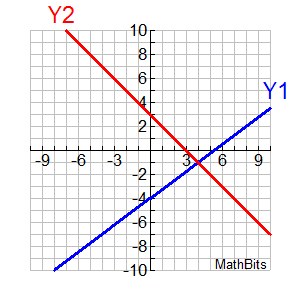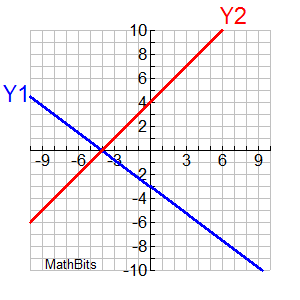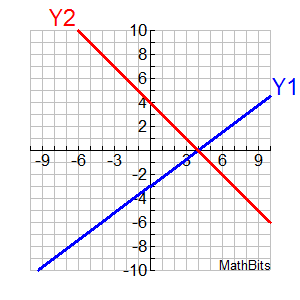8. Given a system of linear equations where the lines each have the same slope. a) Which of the following statements are TRUE regarding the number of possible solutions? Check all that apply, and hit SUBMIT! infinitely many solutions 2 solutions 1 solution no solutions the number of solutions cannot be determined from this information          b) Explain each selection you made.

 9 Solve the following system of equations algebraically.       2a - b = 0       5a - 2b = 3 Show solutions using BOTH methods: "by substitution" and "by elimination".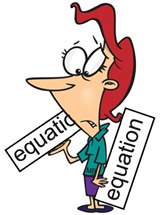10. Solve the following system of equations graphically.